# Community

Blog Events Webinars Tutorials Forum
×
Community Blog Machine Learning Algorithms and Scikit-Learn

# Machine Learning Algorithms and Scikit-Learn

This tutorial looks at the Scikit-Learn library for machine learning and how you can use machine learning algorithms on Alibaba Cloud.

By Harshit Khandelwal, Alibaba Cloud Community Blog author.

This tutorial will focus on the more practical side of the machine learning pipeline. First we will take a look at the Scikit-learn library for machine learning, which is one of the most popular library in machine learning, and then we will give a practical demonstration of machine learning algorithms.

## What Is Scikit-Learn?

Scikit-learn is currently one of the most popular machine learning library in the world. It is easy to use and features several powerful algorithms. It was originally created by David Cournapeau as a 2007 Google summer of code project but later went on to be publically released in 2010 when a team of developers from a research institute in France took the project to new heights. The main language that Scikit-learn is written in is Python, but some of the core algorithms are also written in Cython, which is a combination of C and Python. All of this design helps to ensure the overall good performance of Scikit-learn.

While Scikit-learn is not the only library out there - in fact there are several others and in different languages such as Weka, MLpack, IBM Watson, and TensorFlow - what makes Scikit-learn special is that it was built on top of many other libraries:

• NumPy: base N-dimensional and array package library
• SciPy: library for scientific computing
• Matplotlib: comprehensive 2D plotting library
• IPython: enhanced interactive python console
• Sympy: symbolic mathematics library
• Pandas: data structures and analysis library

More to it, Scikit-learn also provides the following groups of models, all of which are widely used and popular:

• Clustering: One of the most popular type of unsupervised machine learning. It is mainly used for finding hidden patterns and grouping unlabeled data. In the group of these models, the most popular clustering algorithm is K-Means.
• Supervised Models: Probably one of the biggest sets of algorithms present in the Scikit-learn library. Some of the algorithms are: generalized linear models, linear discriminant analysis, naive bayes, lazy packages, neural networks, support vector machines, and decision trees.
• Cross Validation: This can be used for estimating the performance of models, which can be one of the biggest concerns when using such algorithms.
• Datasets: The Scikit-learn library has its own datasets.
• Dimensionality Reduction: This group of models is used for reducing the number of attributes in data for summarization, visualization, and feature selection. Of these, one of the most commonly used algorithms is principal component analysis (PCA).
• Ensemble methods: This group is used for increasing the accuracy of machine learning models.
• Feature extraction: A group of machine learning algorithms for creating new and meaningful features out of the old.
• Feature selection: Selecting a few features from a vast array of features available depending upon some rule.
• Parameter Tuning: Parameters are tuned to get the most out of models.

## How Can I Use Machine Learning Algorithms on Alibaba Cloud?

So now let's see how machine learning works in Alibaba cloud in connection with Scikit-Learn.

The type of machine learning model that you will develop here is a regression algorithm, where prediction is based on continuous numerical values. At the same time, you will integrate other algorithms already found on Alibaba Cloud. Each algorithm will have an evaluation value. Towards the end of the tutorial you will find few sentence description about each algorithm used.

As the first step in this tutorial, obtain some data that's present in the cloud already. For this, I'm using the farmers data.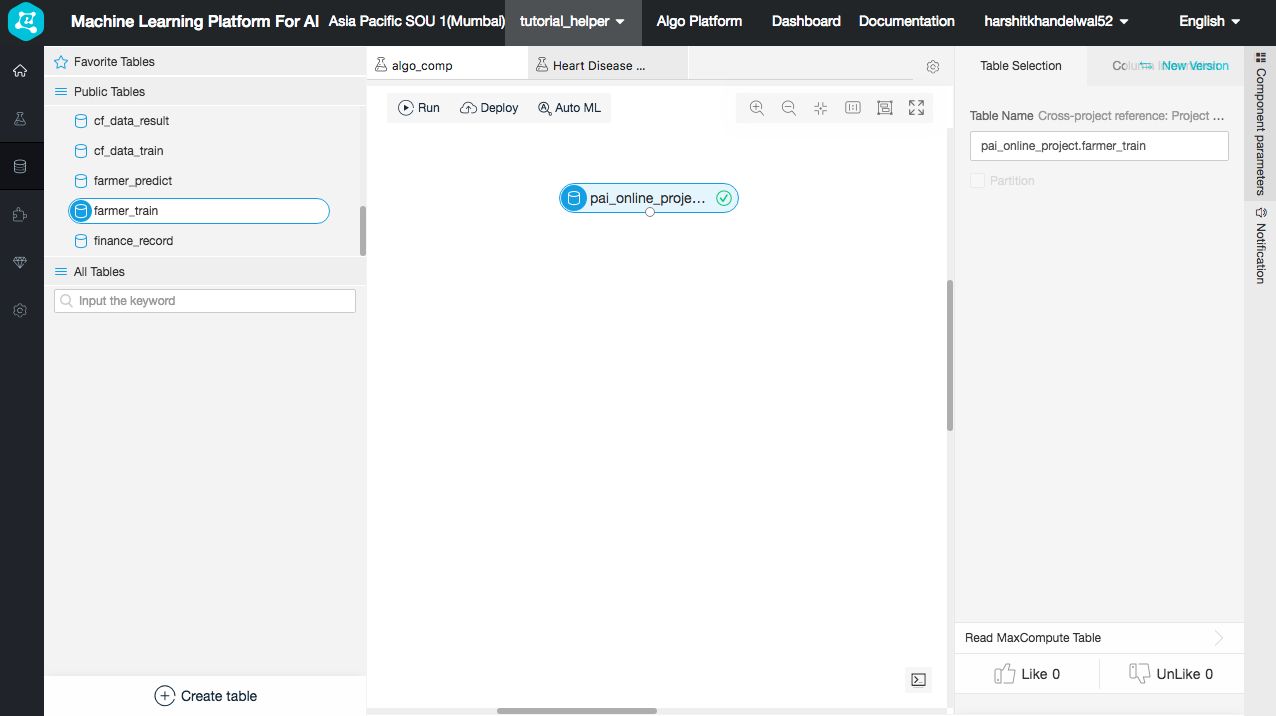The data is as follows: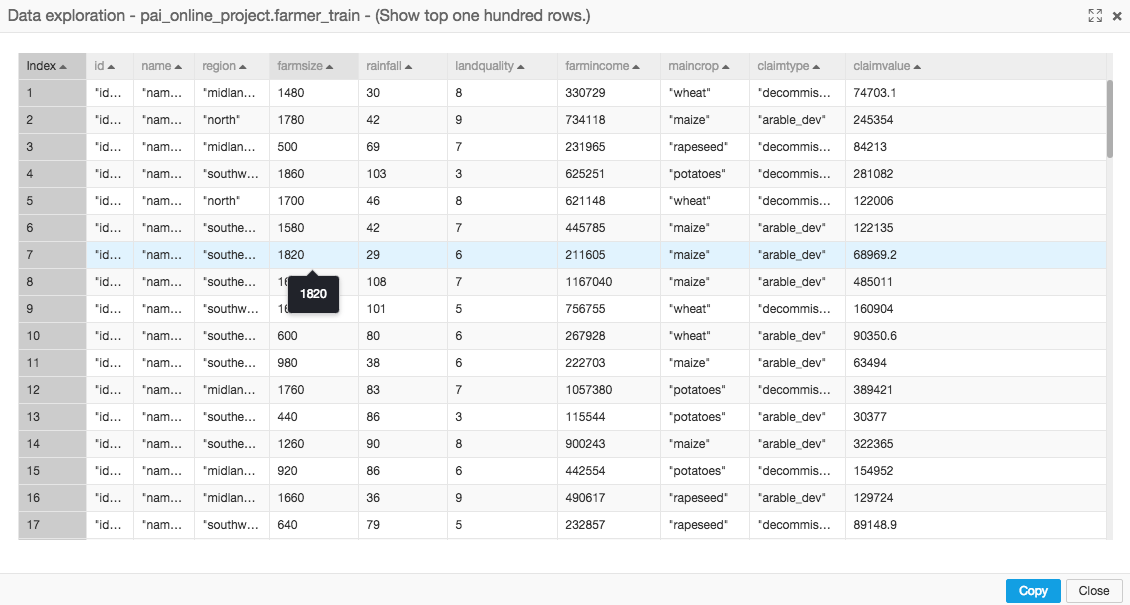As you can see that the data is a mixture of numerical, categorical and string values. However, we want the categorical values into numerical values (because the algorithms we will use only can take numerical values as input and predict numerical values as output). To do this, let's create a SQL script which is imported from the tools column. This script maps the String categorical values to numerical values.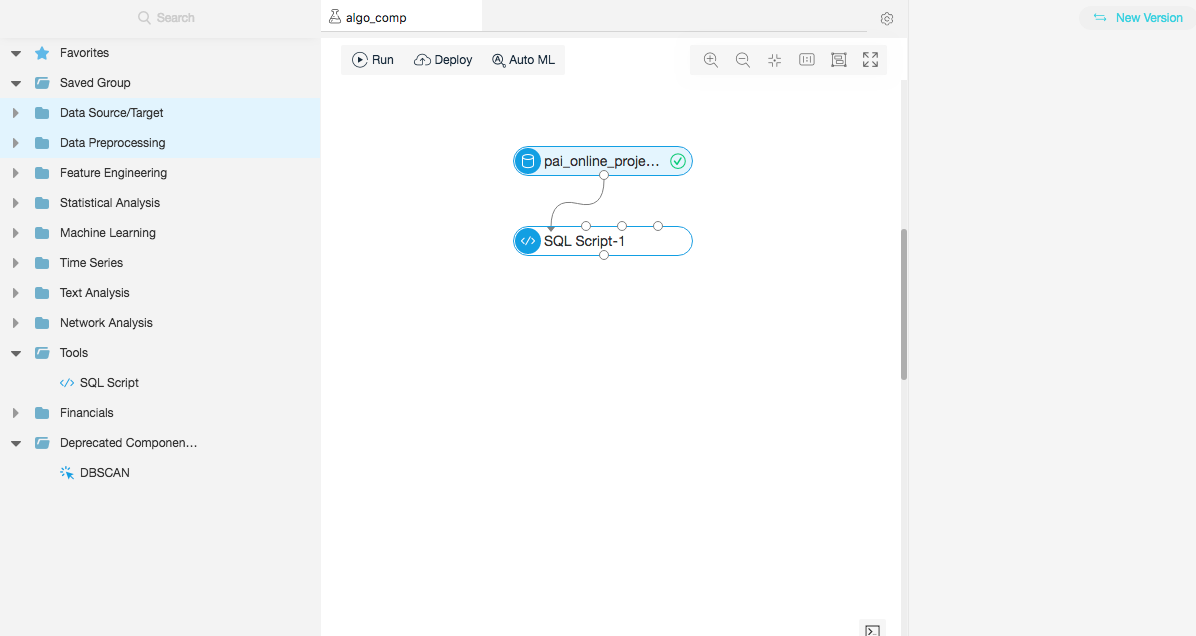What this script does is it takes the columns values and maps each unique value to a number, and the string value gets replaced by numerical value. So, for example, for 'claimtype' column, wherever the script encounters the value 'arable_dev', the script replaces this value with 0 while any other value being encountered by the system gets changed to 1. The Script is as follows: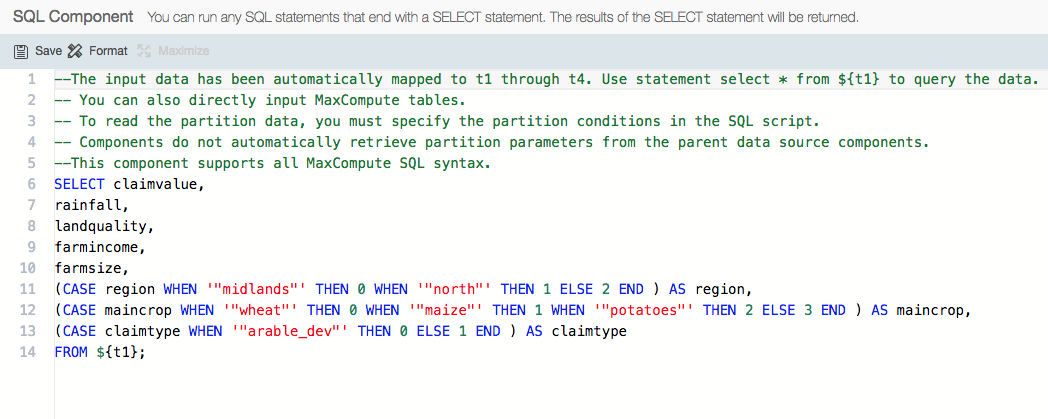Note that the SQL scripts functionally are not only limited to just changing column types, but they can also can be used for combining various data tables into one, combining columns or creating new ones.

Now let's change the data types and normalize the data because the linear regression algorithm can become more biased when values in the column are larger. After that, let's begin with our first machine learning algorithm named Linear Regression.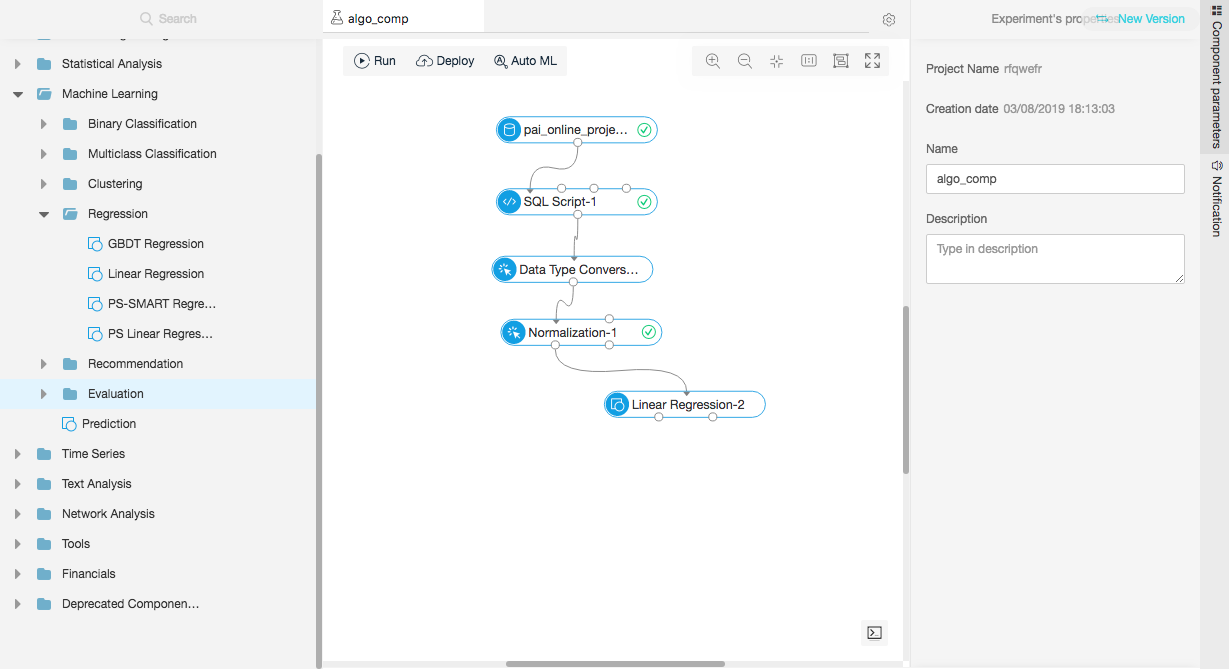Now upon clicking the Linear Regression node, you can select which columns are feature columns and which one is the target column . In this example given in this tutorial, I am using claimvalue column as the target column while all the rest as the features columns. However, because the Linear Regression model has two output nodes, one that gives the result, and the other that gives the analysis report, remember to click the two little check boxes in the end of parameter tab, otherwise the analysis report will not be generated.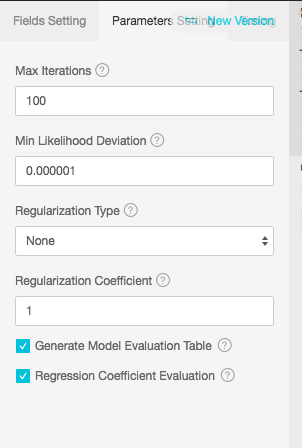You can use more than one linear regression models by just changing the above mentioned parameters and comparing the results for each model and keeping the best while discarding the rest. The analysis report generated from the second output node of the Linear Regression node is as follows. It provides a detailed explanation about how important each column was in the models prediction.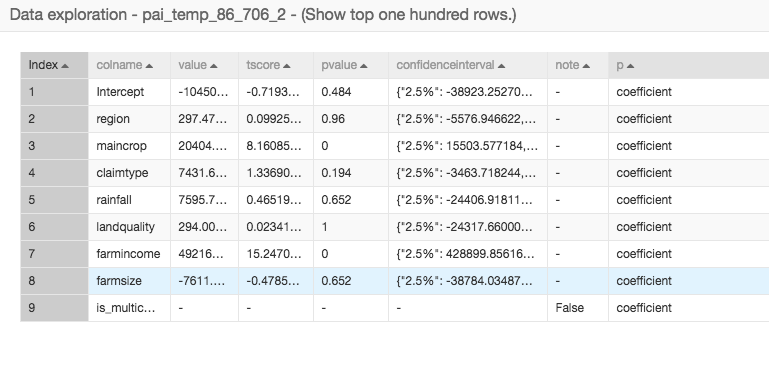Now we continue to use other regression models that are available in the cloud, specifically GBDT regression, PS Linear Regression and PS-SMART Regression. The selection for target and feature columns can be done for each model separately. However, I recommend that you keep each selection similar for each model so that model comparison can be done easily. As you can see, data can be selected from a previous node and extended to more than one node. Alibaba Cloud can run multiple algorithms on same dataset.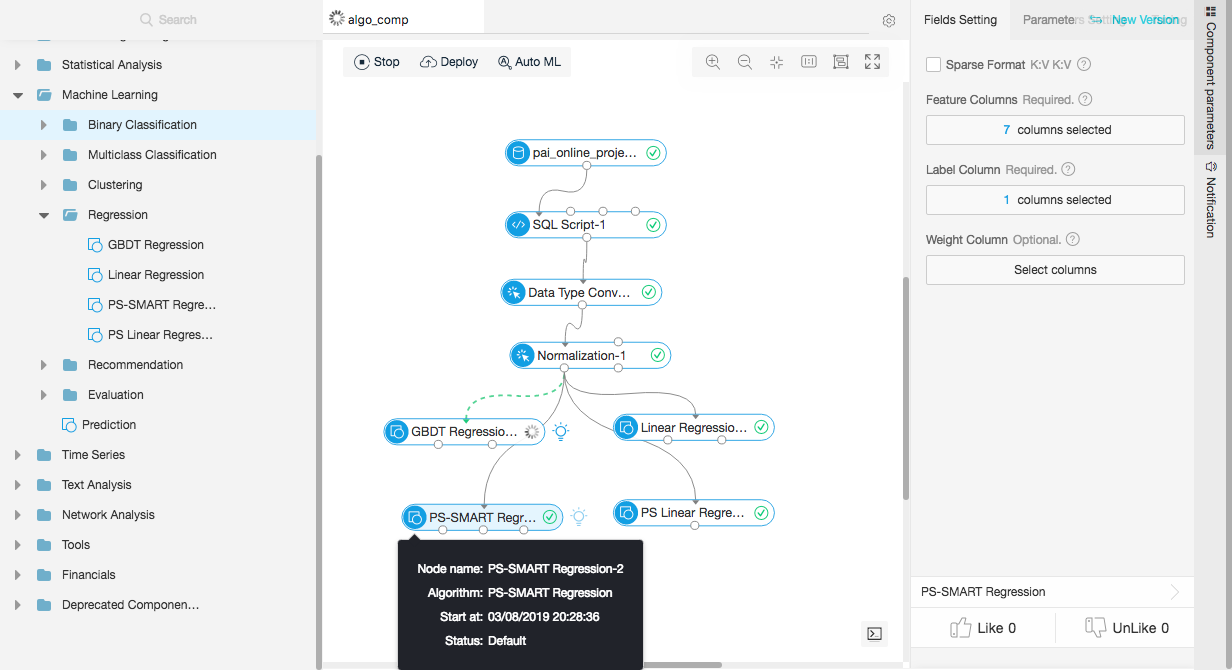Below is the result from PS Linear Regression. You can obtain the results for each model in a similar way.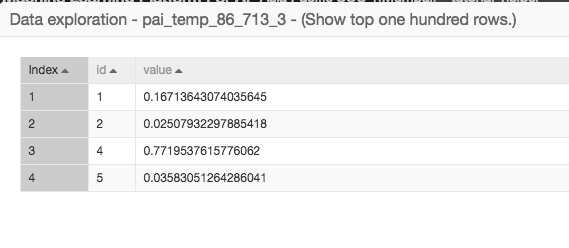So this part of the tutorial covered how you can use various regression algorithms. Now let's explain what else you can do on Alibaba Cloud in connection with the Scikit-learn library for machine learning.

From the image below this, you can all of the machine-learning related algorithms on Alibaba Cloud. In this tutorial, we have already covered regression, so now let's go over classification, which involves predicting binary, ordinal or nominal output. The algorithms that are available for this type are: Random Forest, Logistic Regression, K Nearest Neighbor (KNN), GBDT Classification, along with few others too.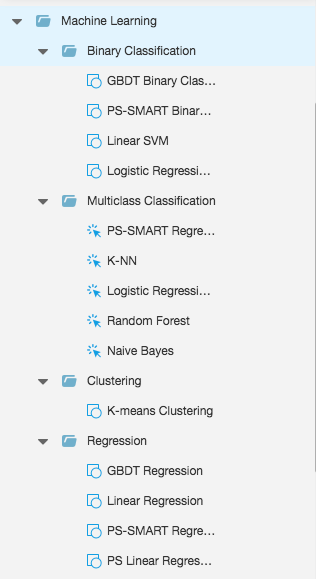Note that, as you can see from the image above, coverage for unsupervised machine learning models on Alibaba Cloud is limited to K means Clustering. However, there are many other unsupervised machine learning models currently under development.

Here's a short explanation of the classification models available on Alibaba Cloud:

• Linear regression: This is one of the earliest developed regression algorithms. ever being developed. This algorithm works similar to the linear equation y=mx+c where x is the input features and the 'y' is the output or target variable.
• Gradient Boosting Decision Tree (GBDT): This algorithm uses a technique called gradient descent to increase the accuracy of results of a machine learning algorithm.
• Parameter Server (PS) Linear Regression: A parameter server (PS) is used to run the online and offline training tasks of large-scale models. Parameter servers can use over a hundred billion rows of samples to train tens of billions of feature models at high efficiency. This algorithm can run training tasks with hundreds of billions of samples and billions of features
• PS-SMART Regression: This also uses a parameter server, which is used for the processing of the online and offline training tasks of large-scale models. In particular, Scalable Multiple Additive Regression Tree (SMART) is an implementation of Gradient boosting decision tree (GBDT) on PS.
0 0 0
Share on

# Related Products

• ##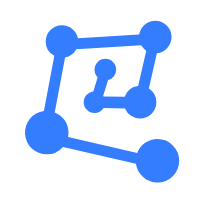Machine Learning Platform For AI

An end-to-end platform that provides various machine learning algorithms to meet your data mining and analysis requirements.

• ##DataWorks

A secure environment for offline data development, with powerful Open APIs, to create an ecosystem for redevelopment.

• ##GPU(Elastic GPU Service)

Powerful parallel computing capabilities based on GPU technology.